# Position, Path Length and Displacement

#### description

• Position - Frame of reference and Reference point
• Distance (Path length)
• Displacement

## Position, Path Length and Displacement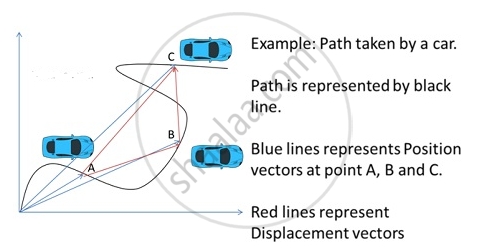The above figure gives the difference between position, path length and displacement

Path Length
Distance travelled by a body is the path length. For example, if a body covers half the circumference of a circle of radius r the distance travelled is d= πr. It is a scalar quantity.

Consider the motion of a car along a straight line from the figure given below. We choose the x-axis such that it coincides with the path of the car’s motion and origin of the axis as the point from where the car started moving, i.e. the car was at x = 0 at t = 0. Let P, Q and R represent the positions of the car at different instants of time. Consider two cases of motion. In the first case, the car moves from O to P. Then the distance moved by the car is OP = +360 m. This distance is called the path length traversed by the car. In the second case, the car moves from O to P and then moves back from P to Q. During this course of motion, the path length traversed is OP + PQ = + 360 m + (+120 m) = + 480 m. Path length is a scalar quantity — a quantity that has a magnitude only and no direction (see Chapter 4).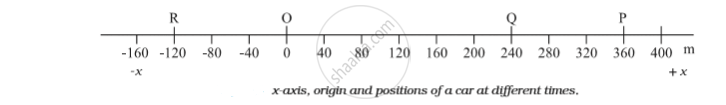Calculating Distance in One-dimensional Motion
Total distance travelled in one-dimension can be found by adding the path lengths for all parts of motion. Note that every path length is greater than 0. Athletes race in a straight track of length 200 m and return back. The total distance travelled by each athlete is 200×2 = 400 m

Displacement Definition
Displacement of the object is equal to the length of the shortest path between the final and the initial points. Its direction is from the initial point to the final point. It is a vector quantity. For example, if a body moves along a circle of radius r and covers half the circumference, then displacement is given by s = 2r.

In one-dimensional motion displacement of the object will be the shortest distance between final and initial point. For example, displacement of a particle in a circular motion would be zero when it reaches the starting point.
The below figure figure is an example of the following:-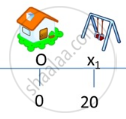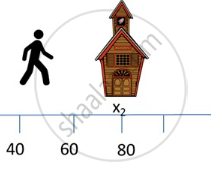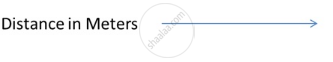If you would like to contribute notes or other learning material, please submit them using the button below.

#### Video Tutorials

We have provided more than 1 series of video tutorials for some topics to help you get a better understanding of the topic.

Series 1

Series 2

### Shaalaa.com

Straight line motion Part 2 (Scalar, vector, distance, displacement) [00:11:20]
S
0%